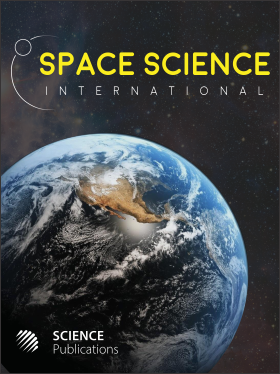Frequency: Continuous
ISSN: 2524-2253 (Print)
ISSN: 2524-2261 (Online)
Research Article Open Access

# Space Time Covariance of Canonical Quantization of Gravity: A (Formal) General Result and the (Rigorous) Explicit Case of 2+1 Quantum Cosmology

T. Christodoulakis1 and G. O. Papadopoulosy1
• 1 University of Athens, Greece

## Abstract

A general classical theorem is presented according to which all invariant relations among the space time metric scalars, when turned into functions on the Phase Space of full Pure Gravity (using the Canonical Equations of motion), be-come weakly vanishing functions of the Quadratic and Linear Constraints. The implication of this result is that (formal) Dirac consistency of the Quantum Operator Constraints (annihilating the wave Function) suffices to guarantee space time covariance of the ensuing quantum theory: An ordering for each invariant relation will always exist such that the emanating operator has an eigenvalue identical to the classical value. The example of 2+1 Quantum Cosmology is explicitly considered: The four possible "Cosmological Solutions" -two for pure Einstein’s equations plus two more when a Λ term is present- are exhibited and the corresponding models are quantized. The invariant relations describing the geometries are explicitly calculated and promoted to operators whose eigenvalues are their corresponding classical values.

Space Science International
Volume 1 No. 2, 2013, 112-128

DOI:

Submitted On: 19 October 2013 Published On: 27 January 2014

How to Cite: Christodoulakis, T. & Papadopoulosy, G. O. (2013). Space Time Covariance of Canonical Quantization of Gravity: A (Formal) General Result and the (Rigorous) Explicit Case of 2+1 Quantum Cosmology. Space Science International, 1(2), 112-128. https://doi.org/10.3844/ajssp.2013.112.128

• 2,320 Views